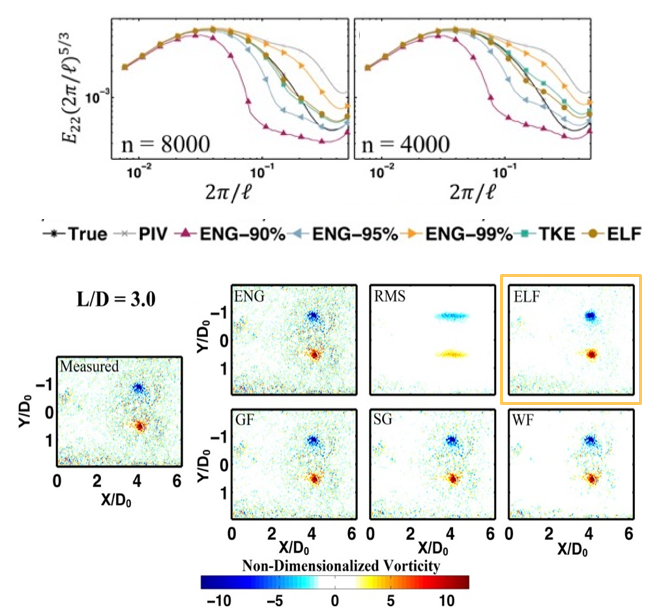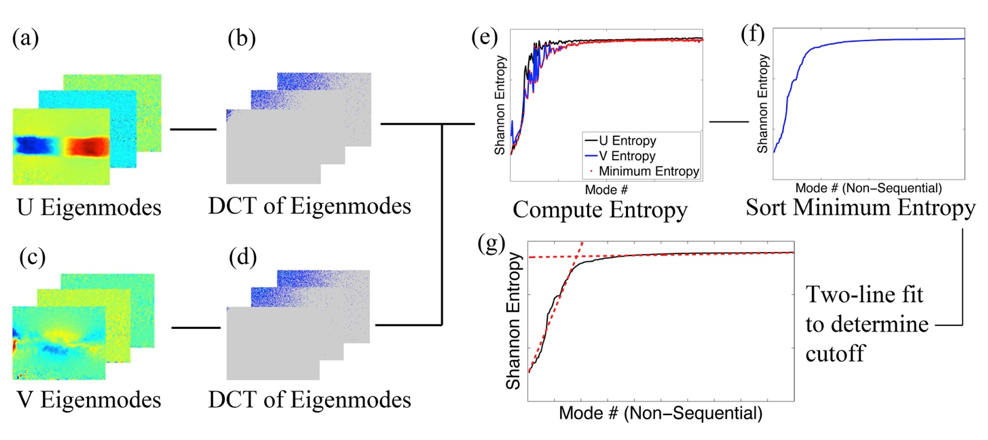Novel autonomous “entropy line-fit” (ELF) thresholding criterion for proper orthogonal decomposition (POD)
17051## 22 Sep Novel autonomous “entropy line-fit” (ELF) thresholding criterion for proper orthogonal decomposition (POD)

Proper orthogonal decomposition (POD) is often used to remove experimental or measurement noise from velocity fields by producing a reduced order model (ROM) of the dataset. The accuracy of POD is primarily governed by the choice of which POD “modes” to use when reconstructing the ROM. If too few modes are maintained, the data will be oversmoothed and lose physical information, while if too many modes are kept, the data will maintain a higher noise level.

The selection of modes is typically done using the energy criterion, where the most energetic modes up to an arbitrarily user-set percentage of the total energy (e.g. 99%) are used. However, the energy criterion is subjective, and energy is not representative of physical information. This issue is most pronounced in transitional and turbulent flows where modes that primarily contain small-scale physical data exist can have similar or even less energy as compared to modes which primarily contain noise.

In this work, we introduce a novel autonomous thresholding method termed the entropy-line fit (ELF) criterion. The ELF method computes the Shannon entropy of the discrete cosine transform (DCT) of each POD mode. Modes are sorted according to their entropy contribution such a set of sequential energy modes may not be kept. The cutoff mode is selected using a two-line fit method.

We tested the ELF criterion using the analytical Hama flow field, synthetic particle image velocimetry (PIV) data produced from homogenous turbulent direct numerical simulation (DNS) flow data, and experimental vortex ring PIV data. The results demonstrate the ELF method excels at differentiating physical data from noise in turbulent flows. Compared to the energy criterion, the ELF criterion produced a more accurate power spectra in the homogenous turbulent flow. Further, the results show the ELF improves the accuracy of post-processing metrics. In the vortex ring data, the ELF criterion yielded circulation values that best matched theoretical expectations.Schematic of the entropy line fit (ELF) thresholding criterion for 2D data. The discrete cosine transform (DCT) of the U and V eigenmodes is computed. The Shannon entropy of the DCT of the eigenmodes is computed and sorted in ascending order. A two-line fit is used to determine the cutoff mode.